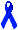Formal Systems and Machines:
An Isomorphism
Peter Suber, Philosophy Department, Earlham College

Formal systems and digital computers are isomorphic. A computer is an instantiation of a formal system, and a formal system is an idealization of a computer. We already know the defining elements of a formal system; here's how they map onto their computer counterparts.

1. The Formal Language. In most computers today each memory address contains one byte (eight bits). Each byte is a series of eight 1's and 0's. Interpreted as numerals in base 2, these bytes code the 256 natural numbers which in base 10 we represent as 0, 1, 2,...255.

But the 1's and 0's are symbols of a formal language. Hence this numerical interpretation is just one among many. Interpreting these bytes under ASCII conventions, they code the Roman alphabet, upper and lower case, the 10 numerals of base 10, the punctuation marks used in English, and other useful (and useless) marks.

The alphabet of the formal language of the computer consists of the 1's and 0's. (Of course, this is just shorthand for speaking of "ons" and "offs", or pulses above and below a critical voltage threshold.)

The grammar that determines which strings of these symbols are wffs consists of one simple rule: any string of eight bits is a wff. Hence each string or numeral from 00000000 to 11111111 is a wff.

For rigor we could say there is a second rule of the grammar: only bytes are wffs. The two rules together say that all and only bytes are wffs.

2. Axioms. The input to the machine plays the role of the axioms. Whether the input comes from the user interactively or from a file, it consists of a set of bytes, or wffs of the formal language. Just as a formal system can have zero axioms, a program can take zero input.

3. Transformation rules. The program running on the machine plays the role of the transformation rules. The program takes the input and transforms it according to the rules coded in the program, and returns some output. Output bytes generated by the program from the input bytes correspond to theorems.

To run a program on a computer is to generate output from the input by means of the rules contained in the program. Input and output are expressed in bytes of bits. In logical terms, this is to generate theorems from axioms by means of rules of inference when the axioms and theorems are expressed as wffs of symbols of the formal language of the system.

 Formal system Digital computer alphabet of the language 1's and 0's (bits) wffs bytes (strings of 8 bits) grammar of the language one rule: all and only bytes are wffs axioms input transformation rules program theorems output proof computation

The consequences of this isomorphism are important for theory.

"Proof" corresponds to "computation". Hence demonstrable limits on provability will translate into demonstrable limits on computability, and vice versa. We'll see this concretely towards the end of the course. For now think about this: what is the machine equivalent of Gödel's first incompleteness theorem? What is the logic-systems equivalent of Turing's proof of the incomputability of the halting problem?

Anything that can be simulated by a computer can be simulated by a formal system, and vice versa. Hence the attempt to write formal systems with "intended interpretations" (e.g. plane geometry, set theory, predicate logic, particle physics, shadow-casting...) is analogous to the attempt to write programs that simulate determinate aspects of reality. From this point of view you can look forward to Gödel's incompleteness theorem and the Löwenheim-Skolem theorem: the first showed that formalizations or simulations of a certain strength always capture less than their intended interpretation, and the second showed they are importantly ambiguous and always capture more than their intended interpretation.

If computers can think, then their machine-states can be isomorphic in relevant ways with brain-states. If so, then these machine- and brain-states will be isomorphic in relevant ways with theorems in some formal system. In this sense "artificial intelligence" is a logical problem, even if "intelligence" is not logical. If the "mind" is reducible to the brain and its states, then our mental life is the output of hardware running software, or equivalently, the instantiation of a formal system. Which formal system?

What is the significance of the fact that computers can be programmed to generate theorems in a formal system? Can formal systems be about themselves? Can machines?

This file is an electronic hand-out for the course, Logical Systems.Peter Suber, Department of Philosophy, Earlham College, Richmond, Indiana, 47374, U.S.A.